# Unit

(diff) ← Older revision | Latest revision (diff) | Newer revision → (diff)

The smallest (positive) natural number:. Multiplication of any number byproduces the same number again.

An elementin a setis called a left (right) unit (left (right) identity) with respect to a binary operationdefined onif for anythe following equation holds: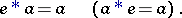If there exists at least one left unit and at least one right unit, then they coincide and there are no other units. If more than one binary operation is defined on(for example, addition and multiplication in a ring), then the term unit is used for only one of these operations, usually multiplication. The unit with respect to addition is called the zero element.

The unit of a lattice is its greatest element, that is, the identity with respect to the operation of intersection (cf. also Lattice).

In an integral domain, any invertible element, that is, an elementthat has an inverse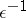such that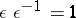, is called a unit, or a divisor of unity. The units of an integral domain form a group under multiplication. The same terminology is sometimes preserved when passing to the field of fractions of the ring(that is, the units ofitself are called units of the field of fractions). For example, the units of an algebraic number fieldare the units of the ring of algebraic integers of, the-adic units are the units of the ring of-adic numbers (cf.-adic number), etc.

The unit morphism (identity morphism) of an objectin a category is the (unique) morphism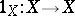satisfying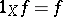and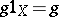for alland all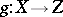.

A standard length when measuring things or comparing objects is called a unit of length, or simply a unit. More generally, various somethings one of whose main parameters is equal to the unit length is called a "unit something" ; e.g. unit vector, unit circle, unit disc, unit cube, unit-cube, unit sphere, unit ball, unit-ball (unit cell), etc.